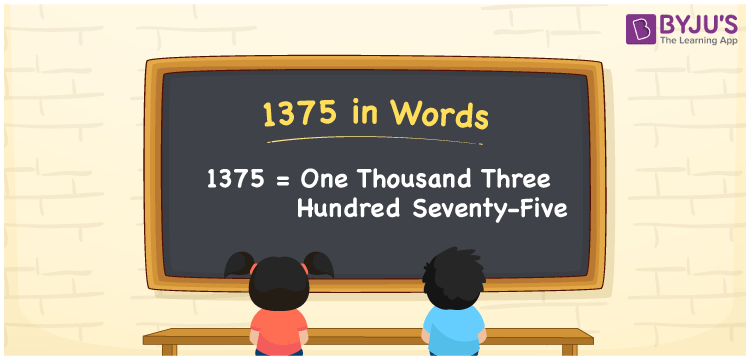# 1375 in words

1375 in words is written as One Thousand Three Hundred and Seventy Five. 1375 represents the count or value. The article on Counting Numbers can give you an idea about count or counting. The number 1375 is used in expressions that relate to money, distance, length, year and others. Let us consider an example for 1375. ”There are One Thousand Three Hundred and Seventy Five steps to reach the temple on a mountain”.

 1375 in words One Thousand Three Hundred and Seventy Five One Thousand Three Hundred and Seventy Five in Numbers 1375

## 1375 in English Words## How to Write 1375 in Words?

We can convert 1375 to words using a place value chart. The number 1375 has 4 digits, so let’s make a chart that shows the place value up to 4 digits.

 Thousands Hundreds Tens Ones 1 3 7 5

Thus, we can write the expanded form as:

1 × Thousand + 3 × Hundred + 7 × Ten + 5 × One

= 1 × 1000 + 3 × 100 + 7 × 10 + 5 × 1

= 1375

= One Thousand Three Hundred and Seventy Five.

1375 is the natural number that is succeeded by 1374 and preceded by 1376.

1375 in words – One Thousand Three Hundred and Seventy Five.

Is 1375 an odd number? – Yes.

Is 1375 an even number? – No.

Is 1375 a perfect square number? – No.

Is 1375 a perfect cube number? – No.

Is 1375 a prime number? – No.

Is 1375 a composite number? – Yes.

## Solved Example

1. Write the number 1375 in expanded form

Solution: 1 × 1000 + 3 × 100 + 7 × 10 + 5 × 1

We can write 1375 = 1000 +300 +70 + 5

= 1 × 1000 + 3 × 100 + 7 × 10 + 5 × 1

## Frequently Asked Questions on 1375 in words

Q1

### How to write 1375 in words?

1375 in words is written as One Thousand Three Hundred and Seventy Five.
Q2

### Is 1375 a perfect square number?

No. 1375 is not a perfect square number.
Q3

### Is 1375 a prime number?

No. 1375 is not a prime number.